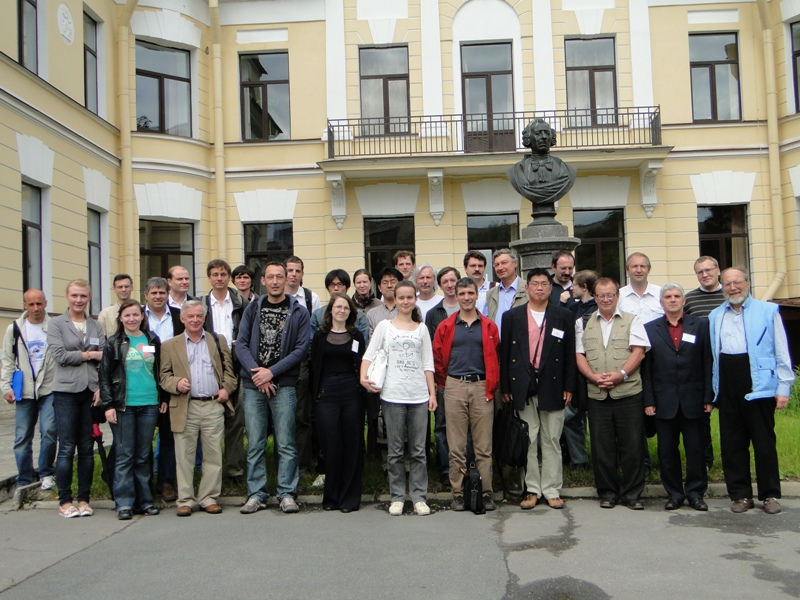### Russia, Saint Petersburg### List of Conference topics:

General ODE equations
Painlevé equations and their generalizations
Painlevé property
Discrete Painlevé equations
Properties of solutions of all mentioned above equations:
• Asymptotic forms and asymptotic expansions
• Connections of asymptotic forms of a solution near different points
• Convergency and asymptotic character of a formal solution
• New types of asymptotic forms and asymptotic expansions
• Riemann-Hilbert problems
• Isomonodromic deformations of linear systems
• Symmetries and transformations of solutions
• Algebraic solutions
Reductions of PDE to Painlevé equations and their generalization ODE systems equivalent to Painlevé equations and their generalizations
Applications of the equations and the solutions